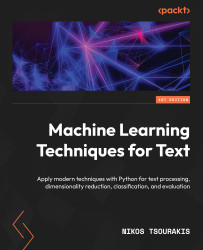•#### Machine Learning Techniques for Text#### Overview of this book

With the ever-increasing demand for machine learning and programming professionals, it's prime time to invest in the field. This book will help you in this endeavor, focusing specifically on text data and human language by steering a middle path among the various textbooks that present complicated theoretical concepts or focus disproportionately on Python code. A good metaphor this work builds upon is the relationship between an experienced craftsperson and their trainee. Based on the current problem, the former picks a tool from the toolbox, explains its utility, and puts it into action. This approach will help you to identify at least one practical use for each method or technique presented. The content unfolds in ten chapters, each discussing one specific case study. For this reason, the book is solution-oriented. It's accompanied by Python code in the form of Jupyter notebooks to help you obtain hands-on experience. A recurring pattern in the chapters of this book is helping you get some intuition on the data and then implement and contrast various solutions. By the end of this book, you'll be able to understand and apply various techniques with Python for text preprocessing, text representation, dimensionality reduction, machine learning, language modeling, visualization, and evaluation.
PrefaceChapter 1: Introducing Machine Learning for TextFree Chapter
Chapter 2: Detecting Spam EmailsChapter 3: Classifying Topics of Newsgroup PostsChapter 4: Extracting Sentiments from Product ReviewsChapter 5: Recommending Music TitlesChapter 6: Teaching Machines to TranslateChapter 7: Summarizing Wikipedia ArticlesChapter 8: Detecting Hateful and Offensive LanguageChapter 9: Generating Text in ChatbotsChapter 10: Clustering Speech-to-Text TranscriptionsIndexOther Books You May Enjoy# Introducing linear regression

Before we delve into solving the main problem of this chapter, we need to provide the necessary theoretical framework. This section presents an ML technique purposely chosen to unfold the discussion and facilitate understanding of the methods that follow.

Let’s consider the three plots in Figure 4.11 that show the relationship between two variables: x and y. In this example, the opaque and transparent points correspond in one of two independent datasets:

Figure 4.11 – Variables with deterministic (A), statistical (B), and random relationship (C)

In Figure 4.11 (A), the points of both datasets reside on their line, which defines a clear deterministic relationship between the two variables. As x changes its value, we can precisely calculate the value of y using one of the line equations. In the middle plot, we cannot predict the exact value of y, but we can obtain a good approximation based again on the line equations...